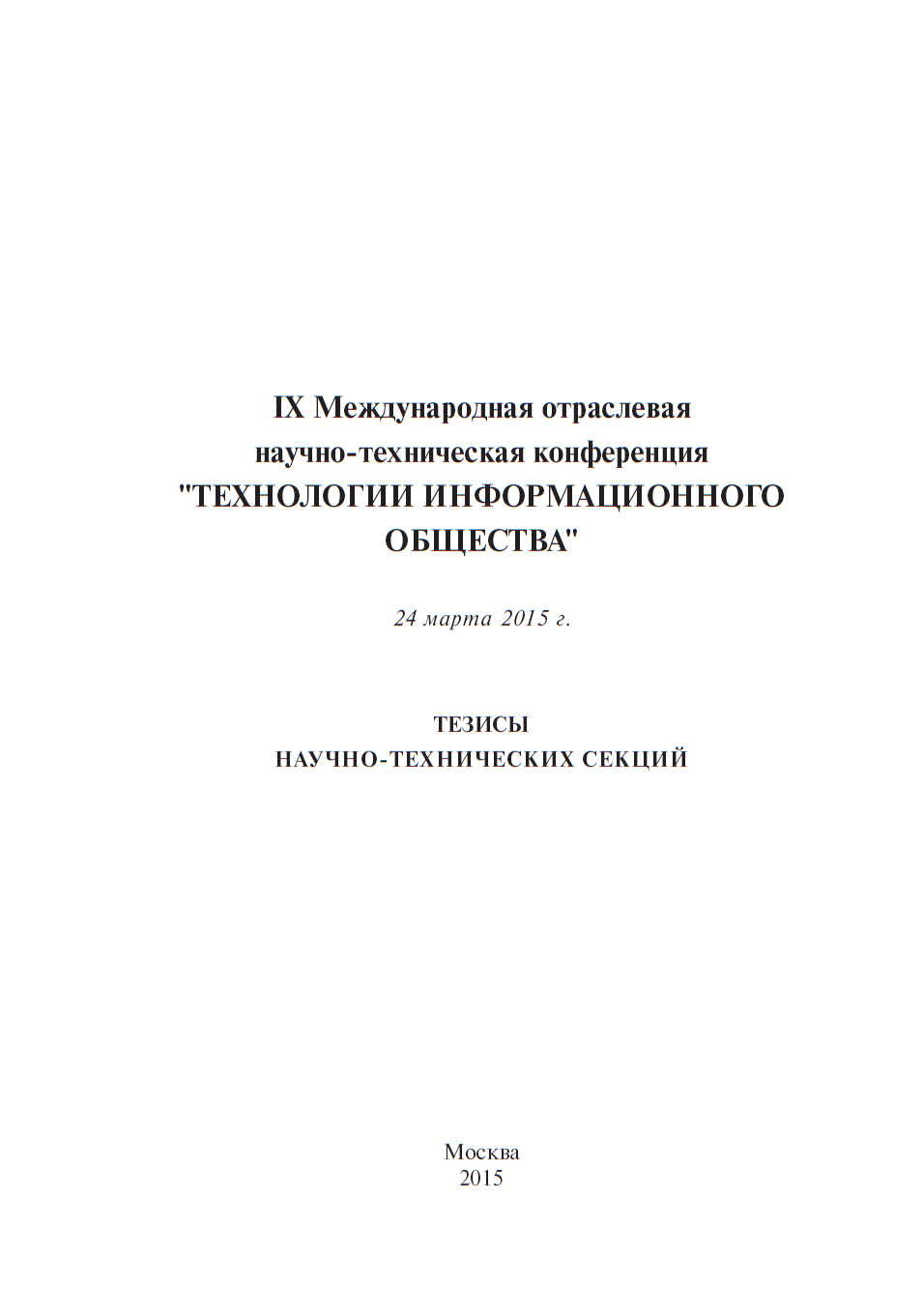• A
• A
• A
• ABC
• ABC
• ABC
• А
• А
• А
• А
• А
Regular version of the site

## Усиление электромагнитных волн миллиметрового диапазона с помощью замедляющих систем типа "петляющий волновод"

С. 83-83.

This paper considers the model of amplification of electromagnetic millimeter waves by non-relativistic electron beams in one-dimensional periodic electrodynamic systems.
As slow-wave structures are investigated systems such as “winding waveguide” and “counter-pins”-type suitable for use in the millimeter range.

The main directions of research are:
-  development of a traveling-wave tube model on the basis of the differential theory of excitation of electrodynamic systems by currents;
-  modeling and calculation for simplified waveguide-resonator model of electrodynamic properties of slow-wave structures such as "winding waveguide" in the millimeter range;
-  representation of a waveguide-resonator model of "winding waveguide"-type  slow-wave structure, composed of segments of rectangular and U-shaped waveguide;
-  obtaining by a waveguide-resonator model coefficients of the transmission matrix, which allows to analyze the dispersion and coupling impedance in the band of amplified frequencies;
-  investigation of “winding waveguide"-type  slow-wave structure taking into account the geometric phase rotation field in neighboring gaps by linear waveguide-resonator model represented by a chain of quadripoles by means of opposite switching of the induced current in the neighboring interaction gaps  and also the first spatial harmonic used in traveling-wave tubes for the calculation of the dispersion;
-  calculation of a number of options that characterize the basic laws of changes in the properties of “winding waveguide"-type  slow-wave structure;
-  modeling the properties of slow-wave structures such as "winding waveguide" using 3D-codes;
-  application of the results obtained using the 3D-codes as the numerical experiment to adjust waveguide-resonator model;
-  model building pin-type slow-wave structures using waveguide-resonator model, customized by experimental reference points.

The paper shows that for modeling slow-wave structures such as “winding waveguide” and “counter-pins” waveguide-resonator model customized to the experimentally obtained reference points can be used. As the reference points can also be used the values of deceleration and the coupling impedance obtained by numerical experiment using HFSS. Waveguide resonator models constructed in such way are sufficiently accurate and simple. This paper shows that these models can be successfully used for the calculation of traveling-wave tubes operating in the millimeter range.

### In bookМ.: ИД Медиа Паблишер, 2015.